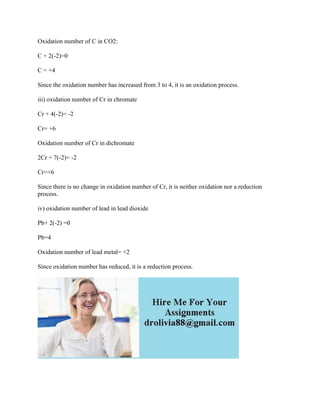Ce diaporama a bien été signalé.
Le téléchargement de votre SlideShare est en cours. ×

# Question T (10 marks) (a) What is the oxidation number of Mn in MnO4(a.docx

Publicité
Publicité
Publicité
Publicité
Publicité
Publicité
Publicité
Publicité
Publicité
Publicité
PublicitéChargement dans…3
×

## Consultez-les par la suite

1 sur 2 Publicité

# Question T (10 marks) (a) What is the oxidation number of Mn in MnO4(a.docx

Question T (10 marks) (a) What is the oxidation number of Mn in MnO4(aq)? (b) Classify the following processes as (A) oxidation, (B) reduction, or (C) neither reduction. You will have to think in terms of oxidation numbers ) FeCla(s) being converted to FeClu(s). (ii) The oxalate ion (COOPlaq) being converted to COS). (Gii) The chromate ion CrO (aq) being converted to the dichromate ion, Cr:O (o). (iv) The formation of metallic lead from lead dioxide.
Solution
a) the total charge on the ion is -1
Oxidation number of Mn + oxidation number of 4 oxygen atoms= -1
Oxidation number of one oxygen atom is -2
Mn + 4(-2)= -1
Oxidation number of Mn = 7
b) i) FeCl2 + 1/2Cl2 ----> FeCl3
Oxidation number of Fe in FeCl2 = -(oxidation number of 2 Cl in FeCl2)
Oxidation number of 2Cl = -2
Oxidation Number of Fe= +2
Oxidation number of Fe in FeCl3= +3
Since oxidation number increases from +2 to +3, it is an oxidation process.
ii) oxidation number of C in oxalate ion:
2C + 2(-2) +2(-2) = -2
C= +3
Oxidation number of C in CO2:
C + 2(-2)=0
C = +4
Since the oxidation number has increased from 3 to 4, it is an oxidation process.
iii) oxidation number of Cr in chromate
Cr + 4(-2)= -2
Cr= +6
Oxidation number of Cr in dichromate
2Cr + 7(-2)= -2
Cr=+6
Since there is no change in oxidation number of Cr, it is neither oxidation nor a reduction process.
Pb+ 2(-2) =0
Pb=4
Oxidation number of lead metal= +2
Since oxidation number has reduced, it is a reduction process.
.

Question T (10 marks) (a) What is the oxidation number of Mn in MnO4(aq)? (b) Classify the following processes as (A) oxidation, (B) reduction, or (C) neither reduction. You will have to think in terms of oxidation numbers ) FeCla(s) being converted to FeClu(s). (ii) The oxalate ion (COOPlaq) being converted to COS). (Gii) The chromate ion CrO (aq) being converted to the dichromate ion, Cr:O (o). (iv) The formation of metallic lead from lead dioxide.
Solution
a) the total charge on the ion is -1
Oxidation number of Mn + oxidation number of 4 oxygen atoms= -1
Oxidation number of one oxygen atom is -2
Mn + 4(-2)= -1
Oxidation number of Mn = 7
b) i) FeCl2 + 1/2Cl2 ----> FeCl3
Oxidation number of Fe in FeCl2 = -(oxidation number of 2 Cl in FeCl2)
Oxidation number of 2Cl = -2
Oxidation Number of Fe= +2
Oxidation number of Fe in FeCl3= +3
Since oxidation number increases from +2 to +3, it is an oxidation process.
ii) oxidation number of C in oxalate ion:
2C + 2(-2) +2(-2) = -2
C= +3
Oxidation number of C in CO2:
C + 2(-2)=0
C = +4
Since the oxidation number has increased from 3 to 4, it is an oxidation process.
iii) oxidation number of Cr in chromate
Cr + 4(-2)= -2
Cr= +6
Oxidation number of Cr in dichromate
2Cr + 7(-2)= -2
Cr=+6
Since there is no change in oxidation number of Cr, it is neither oxidation nor a reduction process.